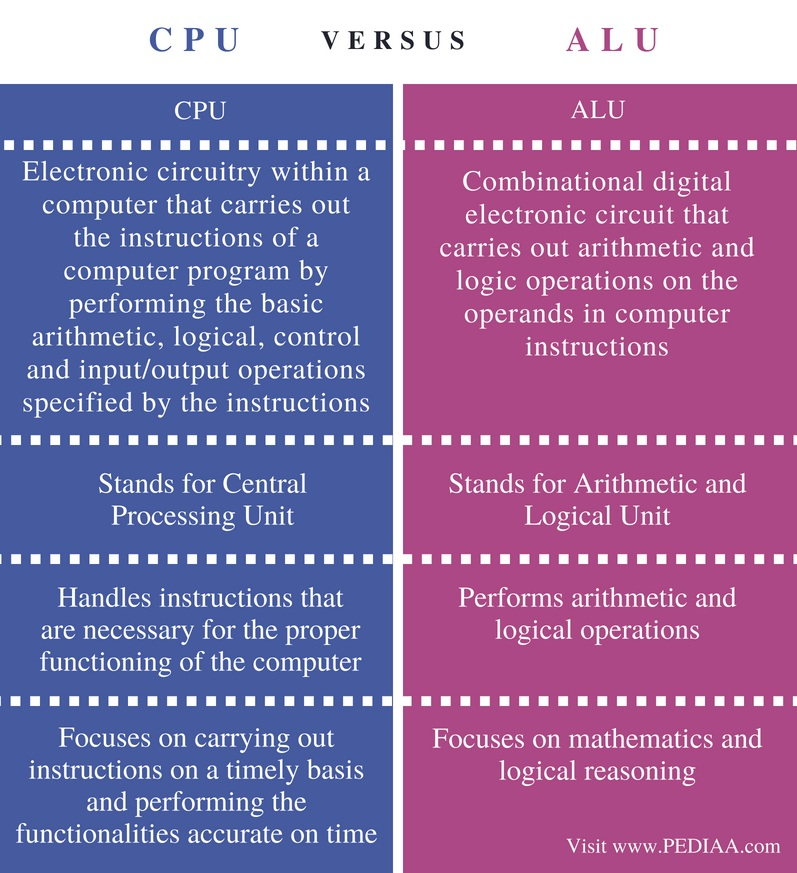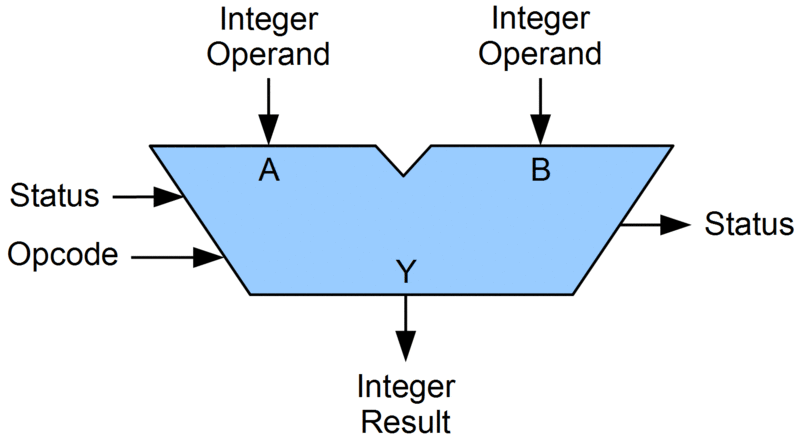# Difference Between CPU and ALU

The main difference between CPU and ALU is that the CPU is an electronic circuit that handles instructions to operate the computer while the ALU is a subsystem of the CPU that performs arithmetic and logical operations.

The most essential component of a computer is the CPU. It carries out instructions to ensure the proper functioning of the computer.  CPU is also known as the processor. ALU and CU are major subcomponents of the CPU. ALU performs arithmetic and logical operations while the CU sends timing and control signals to the other units and manages the resources. CPU depends on system clock, memory, data bus and addresses bus to function properly. In brief, CPU is the central unit of the computer while ALU is a subpart of the CPU.

### Key Areas Covered

1. What is CPU
– Definition, Functionality
2. What is ALU
– Definition, Functionality
3. Relationship Between CPU and ALU
– Outline of Association
4. Difference Between CPU and ALU
– Comparison of Key Differences

### Key Terms

ALU, CPU, Operating System## What is CPU

CPU is the unit in a computer that handles all the functionalities of the computer. It consists of two major components: Arithmetic and Logic Unit (ALU) and the Control Unit(CU). The Arithmetic Unit performs the calculations and logical operations. The CU regulates and synchronizes the tasks of the computer.Figure 1: CPU

Firstly, the CPU fetches the instructions from memory. Then it decodes those instructions to find out the operands and opcode. Finally, that instruction gets executed. The processing unit that performs the actual execution is called the core. Some CPUs consist of multiple cores in a single CPU to execute more instructions simultaneously. A CPU can process a 32bit or 64bit instructions depending on computer architecture. Modern computer systems have multiple CPUs speed up the overall computation speed.

## What is ALU

ALU stands for Arithmetic and Logical Unit and is a subsystem of the CPU. The main objective of ALU is to perform arithmetic and logical operations. Addition, subtraction, division are some examples of arithmetic operations. Logical operations are used to find the logic of the statements such as true or false.

ALUs are also used in Floating Point Units (FPU) and Graphical Processing Units (GPU).Figure 2: ALU

The operation of a simple addition of two integers using the ALU is as follows.  The ALU receives two integer operands. The opcode is the operation performed on operands.  In a mathematical expression “4+3”, 4 and 3 are the operands and the + or the addition is the opcode.  The status register requires information about the previous or the current operation of the ALU. Therefore, there are incoming and outgoing status signals to the ALU. Finally, the ALU will output the result 7.

## Relationship Between CPU and ALU

• ALU is a subsystem of CPU. A single CPU can have multiple ALUs.

## Difference Between CPU and ALU

### Definition

A CPU is the electronic circuitry within a computer that carries out the instructions of a computer program by performing the basic arithmetic, logical, control and input/output operations specified by the instructions. An ALU is a combinational digital electronic circuit that carries out arithmetic and logic operations on the operands in computer instructions.

### Stands for

CPU stands for Central Processing Unit while ALU stands for Arithmetic and Logical Unit.

### Main Functionality

The CPU handles instructions that are necessary for the proper functioning of the computer. On the other hand, ALU performs arithmetic and logical operations.

### Main Focus

CPU focuses on carrying out instructions on a timely basis and performing the functionalities accurate on time.  The ALU focuses on mathematics and logical reasoning.

### Conclusion

The difference between CPU and ALU is that the CPU is an electronic circuit that handles instructions to operate the computer while the ALU is a subsystem of the CPU that performs arithmetic and logical operations. In brief, ALU is a subpart of the CPU.

##### Reference:

1. “Central Processing Unit.” Wikipedia, Wikimedia Foundation, 27 July 2018, Available here.
2. “Arithmetic Logic Unit.” Wikipedia, Wikimedia Foundation, 27 July 2018, Available here.

##### Image Courtesy:

1. “684125” (CC0) via Pixabay
2. “ALU block” By Lambtron – Own work (CC BY-SA 4.0) via Commons Wikimedia